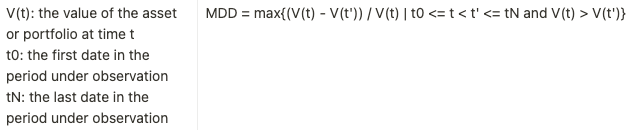# Max drawdown

What is the max drawdown?

The max drawdown of an investment is the greatest percent loss in the investment's value, from its peak to its trough, over a given time period. It is a measure of an investment's downside risk.

What is the formula?What is the step-by-step calculation?

Let's go through the calculation of the max drawdown step-by-step for a symphony backtest.

We'll look at each value in the backtest period, and keep track of two quantities: the maximum value we've seen and the maximum drawdown we've seen, up to that point.

• Start with both the maximum value and the maximum drawdown value set to 0, and consider each day's value in chronological order.
• If a value is greater than the maximum value we've seen so far, record it as such.
• If not, compute the magnitude of the percentage loss between the maximum value we've seen so far, and the value we are observing; this is the drawdown.
• If this drawdown is greater than the maximum drawdown we've seen so far, record it as such.
• Repeat the above steps until we've observed every value.
• The maximum drawdown we've recorded when we've finished observing all the values is the maximum drawdown over the period.